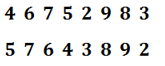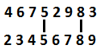Latest Banking jobs   »   Reasoning Ability Quiz For RBI Attendant...

# Reasoning Ability Quiz For RBI Attendant 2021- 02nd March

Directions (1-5): These questions are based on the following set of numbers.

417 843 236 758 625

Q1. If in each number first digit is replaced by the second digit, second digit is replaced by the third digit and third digit is replaced by the first digit, then which number will be the second lowest?
(a) 625
(b) 843
(c) 236
(d) 758
(e) 417

Q2. If in each number, the first and the third digits are interchanged, and then which number will be the highest?
(a) 843
(b) 417
(c) 236
(d) 758
(e) 625

Q3. If all the numbers are arranged in descending order, then what will be the difference between the second digit of highest number and third digit of second highest number?
(a) 1
(b) 2
(c) 4
(d) 5
(e) 0

Q4. If ‘1’ is added to all even digits in all the numbers, in which of the following numbers will the difference between the first and second digit be less than two?
(a) 625
(b) 843
(c) 236
(d) 758
(e) 417

Q5. If in each number all the digits are arranged in ascending order within the number, then what will be the difference between lowest and highest number thus formed?
(a) 431
(b) 469
(c) 530
(d) 433
(e) 429

Q6. If in the digits of the number “46752983” one is added to each even digit and one is subtracted from each odd digit, then how many digits will be repeated in the new number formed?
(a) Two
(b) One
(c) Three
(d) Four
(e) None of these

Q7. If the digits of the number “46752983” are arranged in increasing order form left to right within the number, then how many digits will remain on the same position after the applied operation?
(a) Two
(b) One
(c) Three
(d) Four
(e) None of these

Directions (8-10): The following questions are based on the five three-digit numbers given below:
837 321 215 487 726

Q8. If in each number, all the three digits are arranged in ascending order within the number, which of the following will be the 2nd lowest number?
(a) 487
(b)837
(c) 726
(d)321
(e) 215

Q9. If first digit will be interchanged with second digit, which number will be highest?
(a) 487
(b)837
(c) 726
(d)321
(e) 215

Q10. If 1 is subtracted from the first and 1 is added to the third digits of the numbers , then how many numbers thus formed will be completely divisible by 2?
(a) None
(b) Three
(c) More than three
(d) One
(e) Two

Directions (11-15): These questions are based on the following arrangement. Study it carefully and answer the questions below it.

1 3 5 3 4 5 9 2 8 7 2 3 6 5 2 7 3 8 1 2 1 8 4 9 8 1 2 4 7 3 5 2 4 8 9 8 2 4

Q11. Which element is exactly midway between the seventh element from the left end and sixteenth from the right end?
(a) 8
(b) 2
(c) 5
(d) 6
(e) 7

Q12. How many perfect squares are there to the right of the fourteenth element from the right end?
(a) Two
(b) One
(c) Three
(d) Four
(e) more than four

Q13.How many perfect cubes are there in the above arrangement, each of which is immediately preceded by an odd number and immediately followed by an even number?
(a) None
(b) Three
(c) Two
(d) One
(e) More than three

Q14. How many such odd digits are there in the given arrangement, each of which is immediately followed and preceded by an odd number?
(a) None
(b) One
(c) Two
(d) Three
(e) More than three

Q15. Which of the following element is 5th to the right of 10th from the right end?
(a) 9
(b) 8
(c) 2
(d) 1
(e) 4

Practice More Questions of Reasoning for Competitive Exams:

###### ECGC PO Study Plan 2021

Solutions

S1. Ans.(a)
Sol. New arrangement will be 587 256 174 438 362.

S2. Ans.(d)

S3. Ans.(c)

S4. Ans.(c)

S5. Ans.(a)

S6. Ans(e)
Sol.S7. Ans(a)
Sol.S8.Ans(e)

S9.Ans(a)

S10.Ans(c)

S11.Ans(b)

S12.Ans(e)

S13.Ans(c)

S14.Ans(d)

S15.Ans(b)

Practice with Crash Course and Online Test Series for ECGC PO 2021:×

Thank You, Your details have been submitted we will get back to you.Join India's largest learning destination

What You Will get ?

•Job Alerts
•Daily Quizzes
•Subject-Wise Quizzes
•Current Affairs
•Previous year question papers
•Doubt Solving session

ORJoin India's largest learning destination

What You Will get ?

•Job Alerts
•Daily Quizzes
•Subject-Wise Quizzes
•Current Affairs
•Previous year question papers
•Doubt Solving session

ORJoin India's largest learning destination

What You Will get ?

•Job Alerts
•Daily Quizzes
•Subject-Wise Quizzes
•Current Affairs
•Previous year question papers
•Doubt Solving session

Enter the email address associated with your account, and we'll email you an OTP to verify it's you.Join India's largest learning destination

What You Will get ?

•Job Alerts
•Daily Quizzes
•Subject-Wise Quizzes
•Current Affairs
•Previous year question papers
•Doubt Solving session

Enter OTP

Please enter the OTP sent to
/6

Did not recive OTP?

Resend in 60sJoin India's largest learning destination

What You Will get ?

•Job Alerts
•Daily Quizzes
•Subject-Wise Quizzes
•Current Affairs
•Previous year question papers
•Doubt Solving sessionJoin India's largest learning destination

What You Will get ?

•Job Alerts
•Daily Quizzes
•Subject-Wise Quizzes
•Current Affairs
•Previous year question papers
•Doubt Solving session

Almost there

+91Join India's largest learning destination

What You Will get ?

•Job Alerts
•Daily Quizzes
•Subject-Wise Quizzes
•Current Affairs
•Previous year question papers
•Doubt Solving session

Enter OTP

Please enter the OTP sent to Edit Number

Did not recive OTP?

Resend 60

By skipping this step you will not recieve any free content avalaible on adda247, also you will miss onto notification and job alerts

Are you sure you want to skip this step?

By skipping this step you will not recieve any free content avalaible on adda247, also you will miss onto notification and job alerts

Are you sure you want to skip this step?